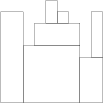You are going to build a stone wall. The wall should be straight and N meters long, and its thickness should be constant; however, it should have different heights in different places. The height of the wall is specified by an array H of N positive integers. H[I] is the height of the wall from I to I+1 meters to the right of its left end. In particular, H is the height of the wall’s left end and H[N−1] is the height of the wall’s right end.

The wall should be built of cuboid stone blocks (that is, all sides of such blocks are rectangular). Your task is to compute the minimum number of blocks needed to build the wall.

Write a function:

``````class Solution { public int solution(int[] H); }
``````

that, given an array H of N positive integers specifying the height of the wall, returns the minimum number of blocks needed to build it.

For example, given array H containing N = 9 integers:

H = 8 H = 8 H = 5 H = 7 H = 9 H = 8 H = 7 H = 4 H = 8 the function should return 7. The figure shows one possible arrangement of seven blocks.Assume that:

N is an integer within the range [1..100,000]; each element of array H is an integer within the range [1..1,000,000,000]. Complexity:

expected worst-case time complexity is O(N); expected worst-case space complexity is O(N) (not counting the storage required for input arguments).

## Code in Java

### Solution-1: StraightForward Solution (Correctness 50%) time-complexity O(n^2)

``````import java.util.*;
import java.util.stream.*;

class Solution {

public int getWalls(int[] H, List<Integer> sortedList) {
int count = 0;
for (int currentMin : sortedList) {
int hLen = H.length;
boolean foundMin = false;
boolean foundCliff = false;
for (int i = 0; i < hLen ;i++) {
//System.out.println("H[i]: " + H[i]);
if (H[i] == 0) {
if (foundMin) {
foundMin = false;
//System.out.println("foundMin is true H[i] is zero: " + i);
count++;
}
}
if (H[i] == currentMin) {
foundMin = true;
H[i] = 0;
}
}
if (foundMin) {
count++;
}
}
return count;
}

public int solution(int[] H) {
List<Integer> intList = IntStream.of(H).boxed().collect(Collectors.toList());
List<Integer> sortedList = intList.stream().distinct().collect(Collectors.toList());
Collections.sort(sortedList);

return getWalls(H, sortedList);
}
}
``````

### Solution-2: Stack Based Solution (Correctness 100%) time-complexity O(n)

``````import java.util.*;
class Solution {
public int solution(int[] H) {
Stack<Integer> stack = new Stack<>();
int hLen = H.length;
stack.push(H);
int count = 0;
for (int i = 1 ; i < hLen; i++) {
int peekVal = stack.peek();
if (peekVal < H[i]) {
stack.push(H[i]);
} else if (peekVal > H[i]) {
stack.pop();
count++;
if (!stack.empty()) {
peekVal = stack.peek();

while (!stack.empty() && peekVal > H[i]) {
count++;
stack.pop();
if (!stack.empty()) {
peekVal = stack.peek();
}
}
if (peekVal == H[i]) {
stack.pop();
}
}
stack.push(H[i]);
}
//System.out.println("stack: " + stack + " count: " + count);
}
count += stack.size();
return count;
}
}

``````